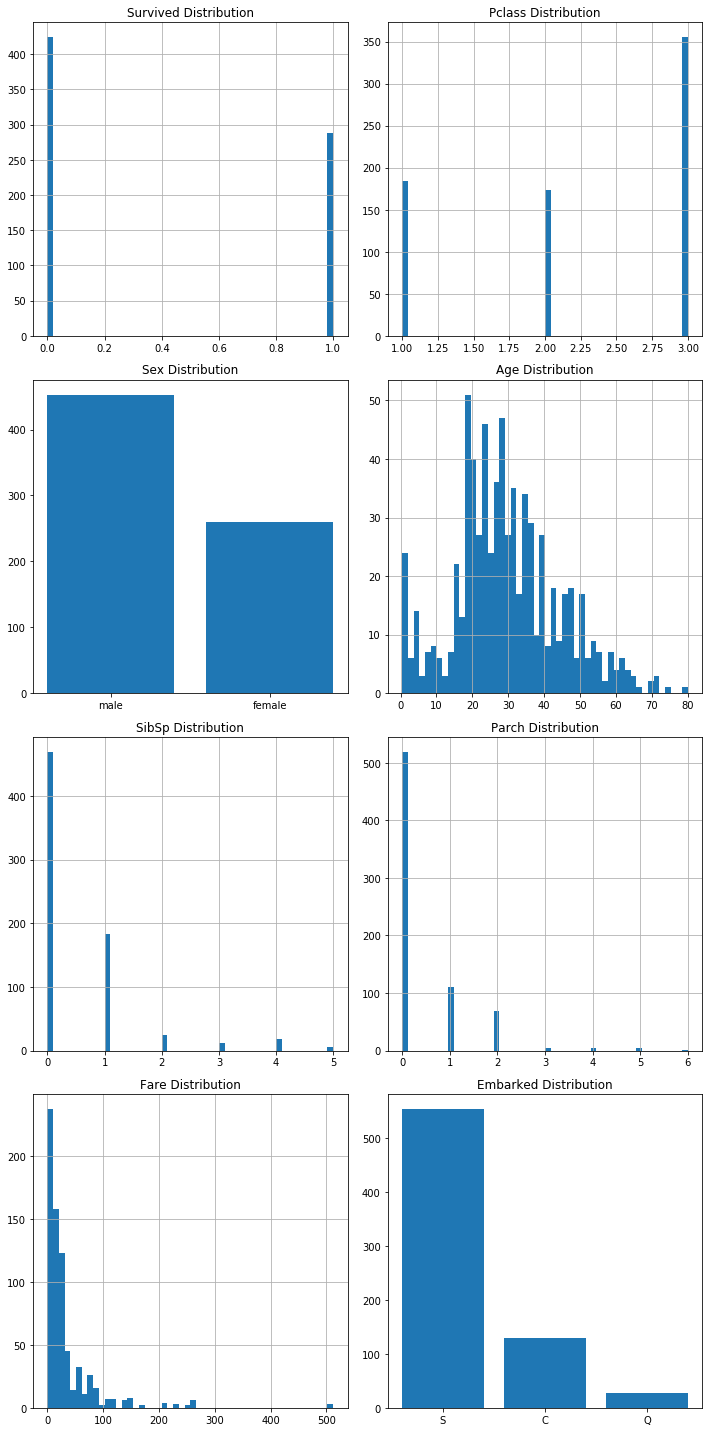#3 Code en Vrac : Neural Network with Tensorflow by RaspVor (Part 1)

Hi reader! Today, on RaspVor.com, let’s make a predictive model by using a Neural Network and Tensorflow. This is really a basis exercise where we will see how to import a CSV database and then modify it in order to use it in a neural network. A lot of tutorial online explain how to predict a signal which can take the value 1 or 0. In order to see something different, we will try to predict a continuous value for instance the fare of a boat ticket.

All the data are completely fake and represent nothing. That’s why the final prediction might not be accurate. There will be several parts and I will try to explain the main principles as much as possible for the different steps of this raspvor code made with python. What is sure is that you have to test the code yourself to better understand.

For the 1st part, we will use a modified version of the titanic database from Kaggle and let’s proceed as we would like to predict the age of the passengers. The results are not good but the goal is mainly to see how to play with a database in python.

Let’s begin !

Import a csv database with Python

The first step is to import the csv. The csv was saved in a folder called « Input » as you can see below:Let’s see the code to import this csv:

In :
#______________________
#|^ ^ ^ ^ ^ ^ ^ ^ ^ ^ ^ ||____
#| The Code!            |||""|""\_,_
#| ____________________l||__|__|__|)
#|(@)@)"""""""'''''**|(@)(@)*****|(@)

#######Upload the csv (input data base)########
import pandas as pd
labeled_data.name = "titanic"

print("Size of the CSV '{0}' : {1}".format(labeled_data.name,labeled_data.shape))
Size of the CSV 'titanic' : (891, 9)

Now the csv is imported, we can manipulate the data. For instance, we will remove the 1st observation which is the Passenger Ids and we will remove all observations with missing values.
This is just an example of manipulation we can make.
For your database, you have to check which observations to delete or to modify (missing values for instance).

In :
########Data and Variables########

#We remove the 1st column "PassengerId"
df = labeled_data.drop('PassengerId', 1)
#We remove the observations with the missing values
df = df.dropna(axis=0)

#Let's "print" the name of variables
list(df)
Out:
['Survived', 'Pclass', 'Sex', 'Age', 'SibSp', 'Parch', 'Fare', 'Embarked']

Study of the database with histograms

We can now study our dataset. In this Raspvor article, we will not perform a full study of the variables. I will just show how to plot in a nice way the histograms for all variables.

In :
################################################
###############Variables Study#############
################################################
In :
########Histograms Part 1########
import numpy as np
import matplotlib.pyplot as plt

#Finding prime number in order to optimize the "plotting" of the histograms
#Finding prime number
def decompose(n):
decompo = np.array([])

i=2
while n>1:
while n%i==0:
decompo = np.concatenate((decompo, np.array([i])))
n=n/i
i=i+1
return decompo

#Automatically define the number of histogram per row and column when we will plot
def axes_x_y(decompo):

x=1
y=1

#loop with a while
i=0
while i < (len(decompo)//2):
x = x * decompo[i]
i += 1

#loop with a for (but for the same kind of result as previously)
for i in range(len(decompo)//2,len(decompo)):
y = y * decompo[i]

return int(x), int(y)
In :
#Let's show exemple of the 2 function above

#1 : we want to find the prime numbers of 8
print("1 : Example - function decompose : {}".format(decompose(8)))

#2 : We want to know, for 8 variables, how many histograms
#we can put per row and column when we use "subplot"
#For instance, if we have 8 variables, we should have 2 histogrames per rows
#and 4 rows.
print("2 : Example - function axes_x_y : {}".format(axes_x_y(decompose(8))))
1 : Example - function decompose : [ 2.  2.  2.]
2 : Example - function axes_x_y : (2, 4)
In :
########Histograms Part 2########
#function to plot the histograms
x, y = axes_x_y(decompose(len(list(df))))

def draw_histograms(df, variables, n_rows, n_cols):
fig=plt.figure(figsize=(10,20))

for i, var_name in enumerate(variables):
if df[var_name].dtype == 'O':
df2 = df[var_name].value_counts()

labels = df2.index.tolist()
indexes = np.arange(len(labels))

plt.bar(indexes, df2)
plt.xticks(indexes, labels)

else:
df[var_name].hist(bins=50,ax=ax)

ax.set_title(var_name+" Distribution")

fig.tight_layout()  # Improves appearance a bit.

plt.show()

#Let's see the result :
draw_histograms(df[df.columns], df.columns, y,x)
#draw_histograms(labeled_data_noIndex[labeled_data_noIndex.columns], labeled_data_noIndex.columns, y,x)In the next RaspVor article, we will see all the transformations we have to perform on the database in order to launch our neural network.